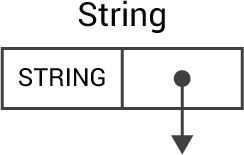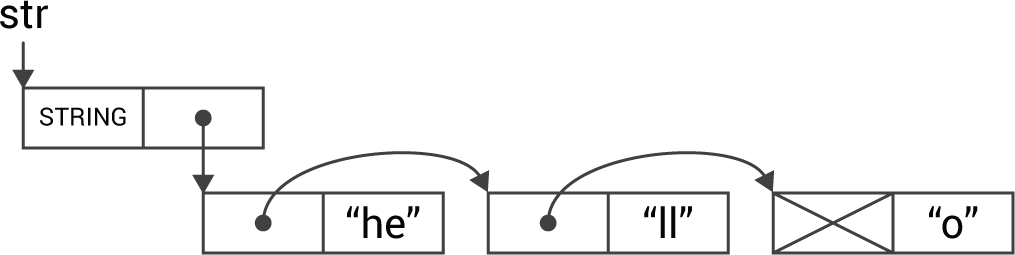## Strings

A string can consist of an arbitrary number of ASCII characters, and the storage required is 2 bytes per character plus four or five bytes. The following section describes how strings are implemented.

Note that in the 32-bit versions of uLisp each object consists of two 4-byte cells, and four ASCII characters are packed into each object.

### String representation

As with all objects in uLisp the head of a string object consists of two 2-byte cells. Strings are identified by the identifier STRING in the left cell, and there is a pointer to the characters in the string in the right cell:A null string just has NULL in the right cell.

In a string of one or more characters the right cell points to a linked list of objects, one object for each pair of characters. This avoids the need to have a separate storage area for strings, and allows strings to be garbage collected in the same way as other objects.

For example, creating a string with:

`(defvar str "hello")`

would give this structure:In a string the cells are linked together using car pointers, rather than the usual cdr pointers, so that the characters won't be affected when the top bit of the car cell is marked during garbage collection.

### Garbage collection

An additional test in markobject() handles the garbage collection of strings:

```void markobject (object *obj) {
MARK:
if (obj == NULL) return;
if (marked(obj)) return;

object* arg = car(obj);
unsigned int type = obj->type;
mark(obj);

if (type >= PAIR || type == ZERO) { // cons
markobject(arg);
obj = cdr(obj);
goto MARK;
}

if (type == STRING) {
obj = cdr(obj);
while (obj != NULL) {
arg = car(obj);
mark(obj);
obj = arg;
}
}
}```

This simply steps along the string, until it reaches a NULL pointer, marking each pair as it goes.

### Reading a string

The utility function readstring() reads in a string up to a specified delimiter and returns the string object:

```object *readstring (uint8_t delim, gfun_t gfun) {
object *obj = newstring();
object *tail = obj;
int ch = gfun();
if (ch == -1) return nil;
while ((ch != delim) && (ch != -1)) {
if (ch == '\\') ch = gfun();
buildstring(ch, &tail);
ch = gfun();
}
return obj;
}```

This calls newstring() to create a string object:

```object *newstring () {
object *ptr = myalloc();
ptr->type = STRING;
ptr->chars = 0;
return ptr;
}```

It then calls buildstring() which allocates a new object for each pair of characters, and then packs the characters into the cdr cell:

```void buildstring (char ch, object **tail) {
object *cell;
if (cdr(*tail) == NULL) {
cell = myalloc(); cdr(*tail) = cell;
} else if (((*tail)->chars & 0xFF) == 0) {
(*tail)->chars = (*tail)->chars | ch; return;
} else {
cell = myalloc(); car(*tail) = cell;
}
car(cell) = NULL; cell->chars = ch<<8; *tail = cell;
}```

### Converting a C string to a Lisp string

The function lispstring() converts a null-delimited C string into a Lisp string and returns it:

```object *lispstring (char *s) {
object *obj = newstring();
object *tail = obj;
char ch = *s++;
while (ch) {
if (ch == '\\') ch = *s++;
buildstring(ch, &tail);
ch = *s++;
}
return obj;
}```

### Printing a string

Finally, the utility function printstring() handles the printing of a string object:

```void printstring (object *form, pfun_t pfun) {
plispstr(form->name, pfun);
}```

The flag PRINTREADABLY is used to determine whether the string is printed with enclosing quotation marks and escape characters, like print, or without them, like princ.

It calls plispstr():

```void plispstr (symbol_t name, pfun_t pfun) {
object *form = (object *)name;
while (form != NULL) {
int chars = form->chars;
for (int i=(sizeof(int)-1)*8; i>=0; i=i-8) {
char ch = chars>>i & 0xFF;
if (tstflag(PRINTREADABLY) && (ch == '"' || ch == '\\')) pfun('\\');
if (ch) pfun(ch);
}
form = car(form);
}
}```

### String functions

Some functions have been added or extended to work with strings:

• The function subseq returns a subsequence of a string.
• The function concatenate joins together an arbitrary number of strings.
• The function string= returns t if its two string arguments are equal, and nil otherwise.
• The function stringp returns t if its argument is a string.
• The function read-line reads in a string up to a return character.
• The function length has been extended to return the number of non-null characters in a string.

Previous: Built-in symbols

Next: Arrays